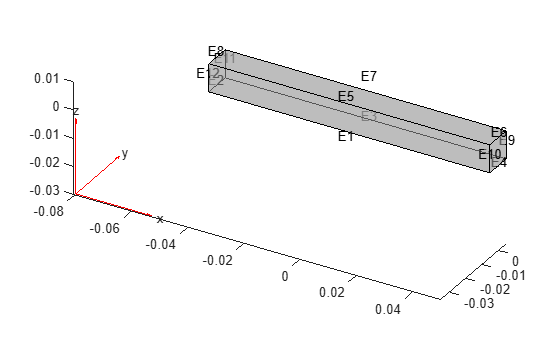# structuralSEInterface

Specify structural superelement interface for component mode synthesis

Since R2019b

## Syntax

``structuralSEInterface(structuralmodel,RegionType,RegionID)``
``sei = structuralSEInterface(___)``

## Description

example

````structuralSEInterface(structuralmodel,RegionType,RegionID)` defines the specified geometric region `RegionType`, `RegionID` as a superelement interface for component mode synthesis. For better performance, specify geometric regions with a minimal number of nodes. For example, use a set of edges instead of a face, or a set of vertices instead of an edge.If you intend to use a reduced-order model in Simscape™ Multibody™, use `structuralBC` instead of `structuralSEInterface`.```
````sei = structuralSEInterface(___)` returns the superelement interface assignment object using the previous syntax. ```

## Examples

collapse all

Define the two ends of the beam as structural superelement interfaces. The reduced-order modeling technique retains the degrees of freedom on these boundaries while condensing all other degrees of freedom.

Create a structural model for modal analysis of a 3-D problem.

`structuralmodel = createpde("structural","modal-solid");`

Create a geometry and include it in the model. Plot the geometry.

```gm = multicuboid(0.1,0.01,0.01); structuralmodel.Geometry = gm; pdegplot(structuralmodel,"EdgeLabels","on","FaceAlpha",0.5)```Specify Young's modulus, Poisson's ratio, and the mass density of the material.

```structuralProperties(structuralmodel,"YoungsModulus",70E9, ... "PoissonsRatio",0.3, ... "MassDensity",2700);```

Generate a mesh.

`generateMesh(structuralmodel);`

Specify the ends of the beam as structural superelement interfaces. For better performance, use the set of edges bounding each side of the beam instead of using the entire face.

```structuralSEInterface(structuralmodel,"Edge",[4,6,9,10]); structuralSEInterface(structuralmodel,"Edge",[2,8,11,12]);```

Reduce the model to all modes in the frequency range `[-Inf,500000]` and the interface degrees of freedom.

`R = reduce(structuralmodel,"FrequencyRange",[-Inf,500000])`
```R = ReducedStructuralModel with properties: K: [166x166 double] M: [166x166 double] NumModes: 22 RetainedDoF: [144x1 double] ReferenceLocations: [] Mesh: [1x1 FEMesh] ```

## Input Arguments

collapse all

Structural model, specified as a `StructuralModel` object. The model contains the geometry, mesh, structural properties of the material, body loads, boundary loads, and boundary conditions.

Example: ```structuralmodel = createpde("structural","transient-solid")```

Geometric region type, specified as `"Vertex"`, `"Edge"`, or, for a 3-D model, `"Face"`.

Example: `structuralSEInterface(structuralmodel,"Face",[2,5])`

Data Types: `char` | `string`

Geometric region ID, specified as a vector of positive integers. Find the region IDs by using `pdegplot`.

Example: `structuralSEInterface(structuralmodel,"Face",[2,5])`

Data Types: `double`

## Output Arguments

collapse all

Superelement interface assignment, returned as a `StructuralSEIAssignment` object. See StructuralSEIAssignment Properties.

## Version History

Introduced in R2019b